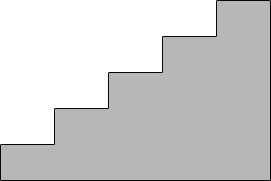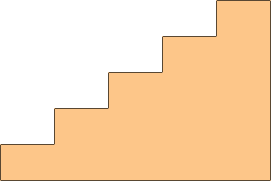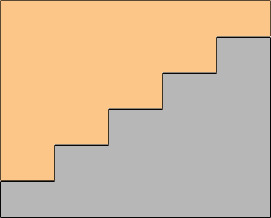SEARCH HOMEMath Central Quandaries & QueriesQuestion from jacques, a student: A football stand 150 yd long has 20 tiers of seats; each tier has a rise of 2ft and tread of 3ft.Its construction of reinforced concrete with a cross section as shown. Find the amount of the material used in its construction.Jacques,

You didn't send a drawing of the cross section but I expect it looks like this. (Mine only has 5 tiers.)The volume of concrete needed is the area of the cross section times the length of the stand which is 150 yards. To find the area of the cross section I would draw a second copy,and then turn it 180 degrees and put it on top of the first copy.What is the area of this rectangle?

PennyMath Central is supported by the University of Regina and The Pacific Institute for the Mathematical Sciences.